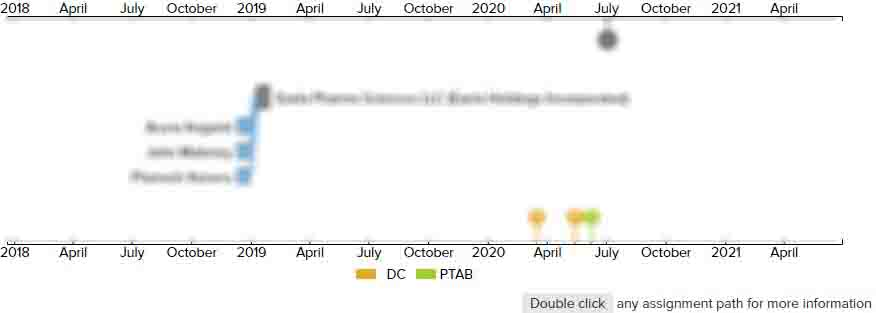# Document comparison using multiple similarity measures

• US 7,472,121 B2
• Filed: 12/15/2005
• Issued: 12/30/2008
• Est. Priority Date: 12/15/2005
• Status: Expired due to Fees
##### First Claim
Patent Images

1. A method of comparing a plurality of documents, said method comprising:

• determining a plurality of similarity measures for said plurality of documents; and

determining an overall similarity measure for said plurality of documents, based on said plurality of similarity measures,wherein said plurality of similarity measures are chosen from the group of similarity measures comprising;

a semantic similarity measures based on similarity of terms contained in said plurality of documents;

a structural similarity measure based on the structures of chemical terms described in said plurality of documents; and

a reference similarity measure based on references contained in said plurality of documents;

wherein said plurality of similarity measures include a semantic similarity measure and a reference similarity measure, andfurther wherein two documents Pi and Pj being compared are represented by corresponding vectors;

di={wi1, wi2, . . . , win}, where wik (1<

k<

n) is a non-negative value denoting the weight of the term k in the document i, anddj={wj1, wj2, . . . , wjn}, where wil (1<

l<

n) is a non-negative value denoting the weight of the term l in the document j,and the semantic similarity measure SemSim( ) is given by the equation;

in which where di·

dj is the dot product between the vectors, calculated asΣ

(from k=0 to n)Σ

(from 1=0 to n) wik·

wil;

and wherein if terms k and l belong to ontology classes Ck and Cl, respectively, wik·

wil is calculated as;

tfik×

tfjl×

idf(Ck)×

idf(Cl)×

sim(Ck, Cl)×

WTont , wherein tfik is the frequency of the term k in the document i, tfjl is the frequency of the term l in the document j, idf(Ck) represents the inverse document frequency of class Ck in document corpus of document i and is calculated as;

log2 (N/nCk), where N is the number of documents in a collection, and nCk is the number of documents in which a term of class Ck occurs at least once, idf(Cl) represents the inverse document frequency of class Cl in document corpus of document j and is calculated as;

log2 (N/nCl) , where N is the number of documents in a collection, and nCl is the number of documents in which a term of class Cl occurs at least once, sim(Ck, Cl) is calculated as;

where pCk is the probability of encountering the class Ck or a child of the term in a given taxonomy, pCl is the probability of encountering the class Cl or a child of the term in a given taxonomy and pmin is the minimum probability among common ancestors of classes Ck and Cl, andWTont is a predefined constant in the range 0<

WTont<

1.

• ##### 1 Assignment
Timeline View
Assignment View×
×